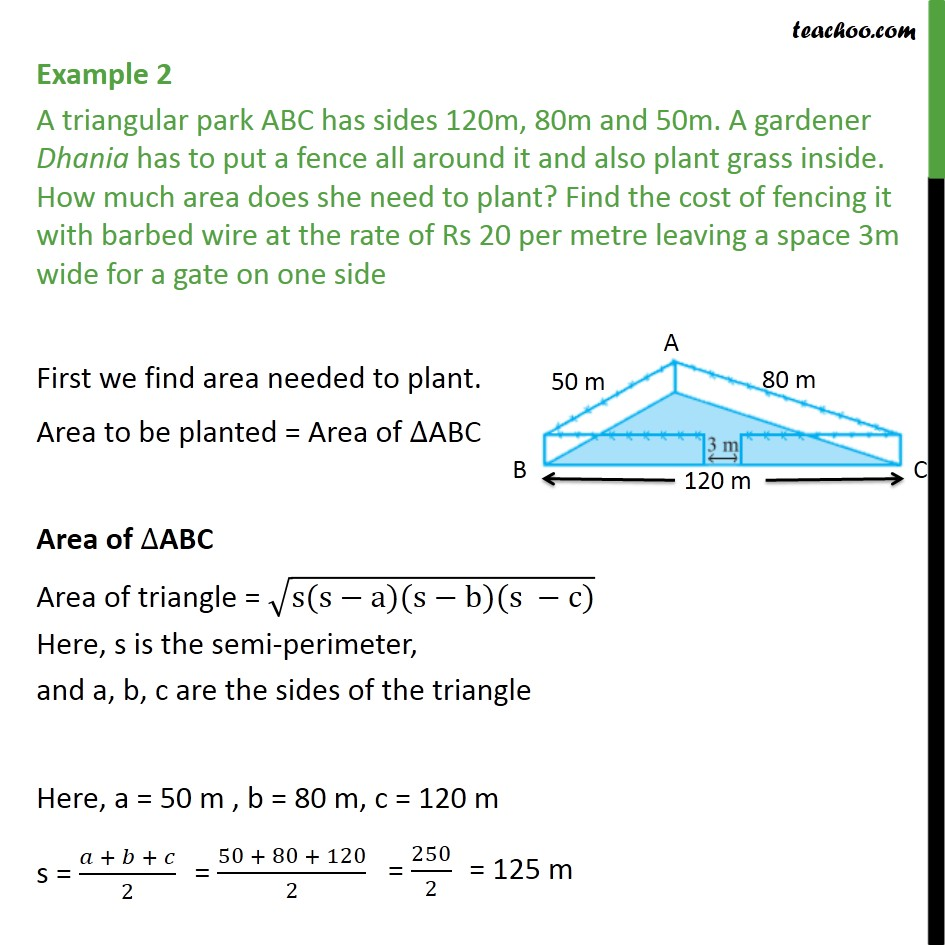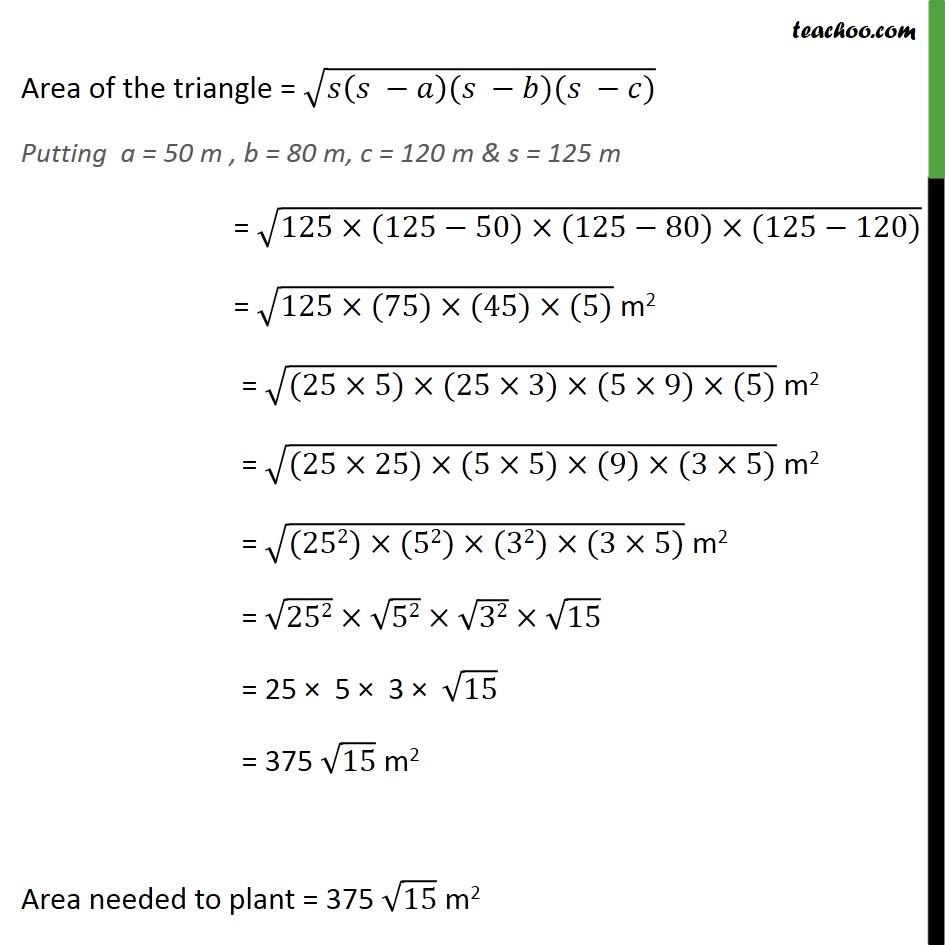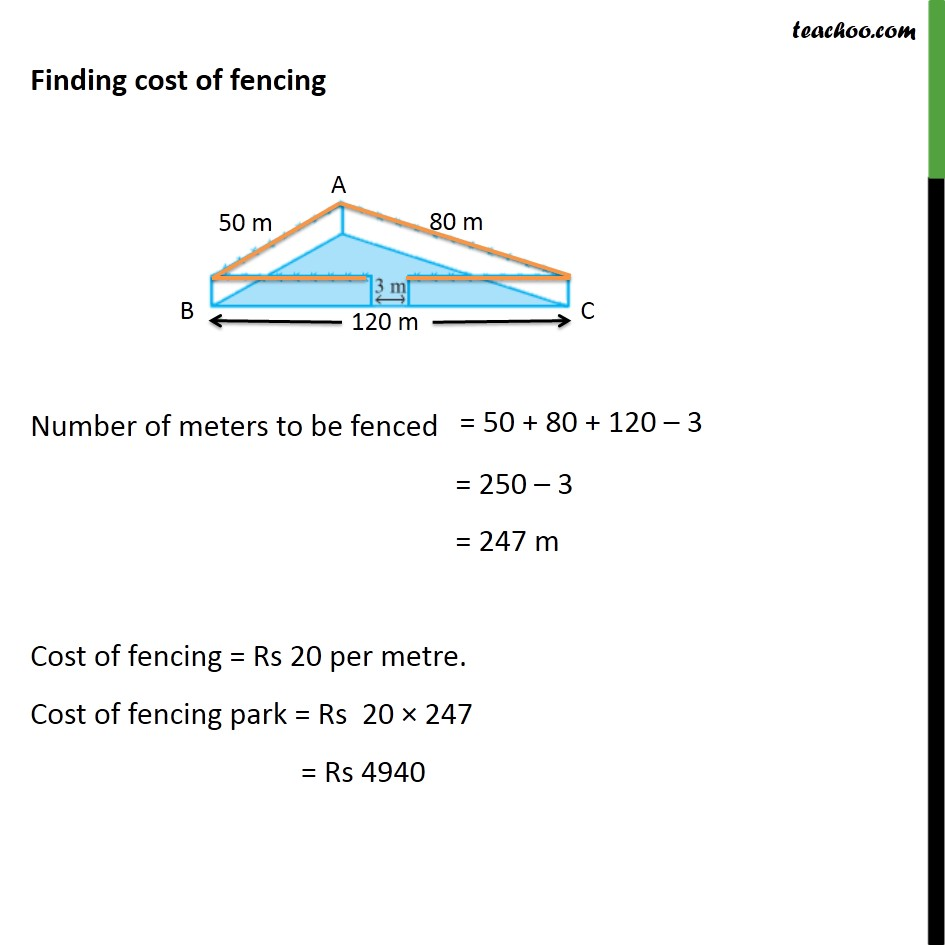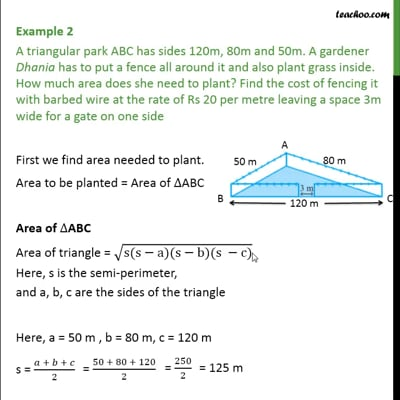Chapter 12 Class 9 Herons Formula

Class 9
Important Questions for Exam - Class 9This video is only available for Teachoo black users

Introducing your new favourite teacher - Teachoo Black, at only ₹83 per month

### Transcript

Example 2 A triangular park ABC has sides 120m, 80m and 50m. A gardener Dhania has to put a fence all around it and also plant grass inside. How much area does she need to plant? Find the cost of fencing it with barbed wire at the rate of Rs 20 per metre leaving a space 3m wide for a gate on one side First we find area needed to plant. Area to be planted = Area of ABC Area of ABC Area of triangle = (s(s a)(s b)(s c)) Here, s is the semi-perimeter, and a, b, c are the sides of the triangle Here, a = 50 m , b = 80 m, c = 120 m s = ( + + )/2 Area of the triangle = ( ( )( )( )) Putting a = 50 m , b = 80 m, c = 120 m & s = 125 m = (125 (125 50) (125 80) (125 120)) = (125 (75) (45) (5)) m2 = ((25 5) (25 3) (5 9) (5)) m2 = ((25 25) (5 5) (9) (3 5)) m2 = ((252) (52) (32) (3 5)) m2 = 252 52 32 15 = 25 5 3 15 = 375 15 m2 Area needed to plant = 375 15 m2 Finding cost of fencing Number of meters to be fenced = 250 3 = 247 m Cost of fencing = Rs 20 per metre. Cost of fencing park = Rs 20 247 = Rs 4940